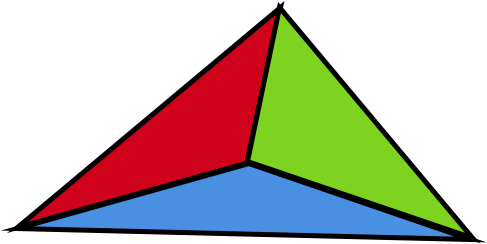# It's All Good

Geometry Level 2Let $O=(0,0), P=(3,4), Q=(6,0)$ be the vertices of triangle $OPQ.$ Point $R$ inside the triangle is such that triangles $OPR, PQR, OQR$ are of equal area.

The product of the coordinates of $R$ is $\text{\_\_\_\_\_\_\_\_\_\_}.$

×